# On necessary and sufficient cryptographic assumptions the case

• Slides: 52
Download presentationOn necessary and sufficient cryptographic assumptions: the case of memory checking Lecture 2 : Authentication and Communication Complexity Lecturer: Moni Naor Weizmann Institute of Science Web site of lectures: www. wisdom. weizmann. ac. il/~naor/COURSE/ens. htmlRecap of Lecture 1 • Key idea of cryptography: use computational intractability for your advantage • One-way functions are necessary and sufficient to solve the two guard identification problem – Notion of Reduction between cryptographic primitives • Equivalence of the existence of one-way functions:Existence of one-way functions is equivalent: The existence of one-way functions is equivalent to • Pseudo-random generators [HILL] • Pseudo-random functions and permutations – Block ciphers • Bit commitment – Implies zero-knowledge • Signature Schemes • (Non trivial) shared-key encryption Goal of these talk: add two other items to the list: • Sub-linear authentication • Memory CheckingAuthentication • Verifying that a string has not been modified – A central problem in cryptography – Many variants • Relevant both in communication and in storageThe authentication problem one-time version • Alice would want to send a message m {0, 1}n to Bob • They want to prevent Eve from interfering – Bob should be sure that the message m’ he receives is equal to the message m Alice sent m Alice Eve Bob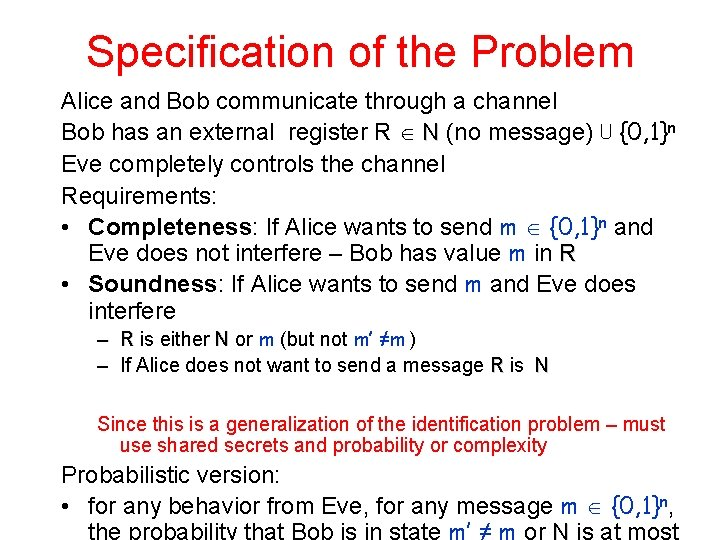Specification of the Problem Alice and Bob communicate through a channel Bob has an external register R N (no message) ⋃ {0, 1}n Eve completely controls the channel Requirements: • Completeness: If Alice wants to send m {0, 1}n and Eve does not interfere – Bob has value m in R • Soundness: If Alice wants to send m and Eve does interfere – R is either N or m (but not m’ ≠m ) – If Alice does not want to send a message R is N Since this is a generalization of the identification problem – must use shared secrets and probability or complexity Probabilistic version: • for any behavior from Eve, for any message m {0, 1}n,Authentication using hash functions • Suppose that – H= {h| h: {0, 1}n → {0, 1}k } is a family of functions – Alice and Bob share a random function h H – To authenticate message m {0, 1}n Alice sends (m, h(m)) – When receiving (m’, z) Bob computes h(m’) and compares to z • If equal, moves register R to m’ • If not equal, register R stays in N • What properties do we require from H – hard to guess h(m’) - at most ε • But clearly not sufficient: one-time pad. – hard to guess h(m’) even after seeing h(m) - at most ε • Should be true for any m’ – Short representation for h - must have small log|H|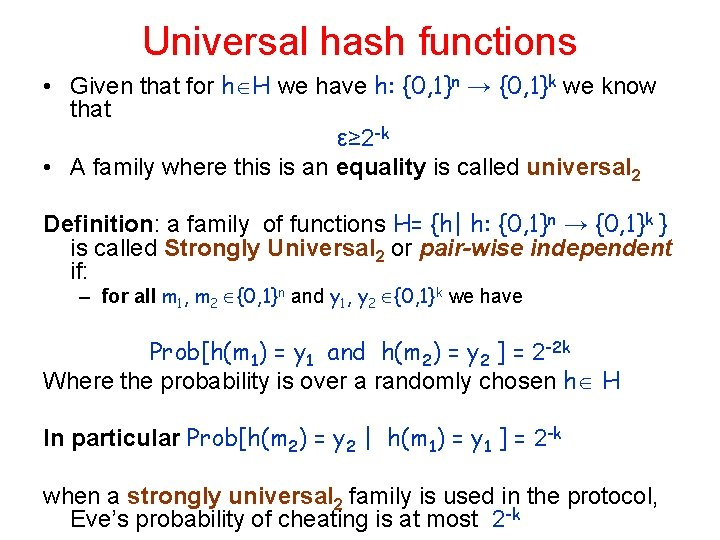Universal hash functions • Given that for h H we have h: {0, 1}n → {0, 1}k we know that ε≥ 2 -k • A family where this is an equality is called universal 2 Definition: a family of functions H= {h| h: {0, 1}n → {0, 1}k } is called Strongly Universal 2 or pair-wise independent if: – for all m 1, m 2 {0, 1}n and y 1, y 2 {0, 1}k we have Prob[h(m 1) = y 1 and h(m 2) = y 2 ] = 2 -2 k Where the probability is over a randomly chosen h H In particular Prob[h(m 2) = y 2 | h(m 1) = y 1 ] = 2 -k when a strongly universal 2 family is used in the protocol, Eve’s probability of cheating is at most 2 -kConstructing universal hash functions The linear polynomial construction: • fix a finite field F of size at least the message space 2 n – Could be either GF[2 n] or GF[P] for some prime P ≥ 2 n • The family H of functions h: F→ F is defined as H= {ha, b(m) = a∙m + b | a, b F} Claim: the family above is strongly universal 2 Proof: for every m 1, m 2 , y 1, y 2 F there are unique a, b F such that a∙m 1+b = y 1 a∙m 2+b = y 2 Size: each h H represented by 2 n bitsLower bound on size of strongly universal hash functions Theorem: let H= {h| h: {0, 1}n → {0, 1} } be a family of pair-wise independent functions. Then |H| is Ω(2 n) More precisely, to obtain a d-wise independence family |H| should be Ω(2 n└d/2┘) Theorem: see N. Alon and J. Spencer, The Probabilistic Method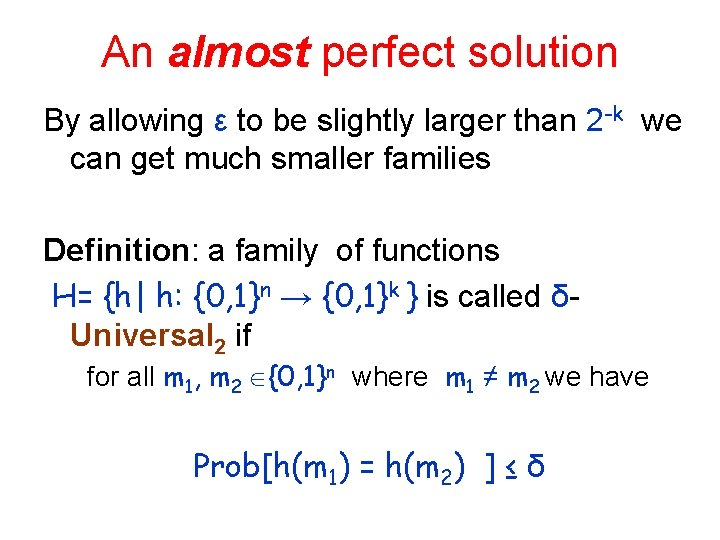An almost perfect solution By allowing ε to be slightly larger than 2 -k we can get much smaller families Definition: a family of functions H= {h| h: {0, 1}n → {0, 1}k } is called δUniversal 2 if for all m 1, m 2 {0, 1}n where m 1 ≠ m 2 we have Prob[h(m 1) = h(m 2) ] ≤ δAn almost perfect solution Idea: combine • a family of δ-Universal 2 functions H 1= {h| {0, 1}n → {0, 1}k } with • a Strongly Universal 2 family H 2= {h| {0, 1}k → {0, 1}k } Consider the family H where each h H is {0, 1}n → {0, 1}k is defined by h 1 H 1 and h 2 H 2 h(x) = h 2(h 1(x)). As before Alice sends m, h(m) Claim : probability of cheating is at most δ + 2 -k Proof: when Eve sends m’, y’ we must have m ≠ m‘ but either – y’ =h(m), which means that Eve succeeds with probability at most δ + 2 -k • Collision in h 1 Or in h 2 Or – y’ ≠ h(m) which means that Eve succeeds with probability at most 2 -k • Collision in h 2 Size: each h H represented by log |H 1 |+ log |H 2|Constructing almost universal hash functions The polynomial evaluation construction {0, 1}n → {0, 1}k : • fix a finite field F of size at least the target space 2 k – Could be either GF[2 k] or GF[P] for some prime P ≥ 2 k • Let n = ℓ ∙ k • Treat each (non-zero) message m {0, 1}n as a degree (ℓ 1)- polynomial over F. Denote by Pm m • The family H of functions h: Fℓ → F is defined by all elements in F: H= {hx (m)= Pm (x)| x F} Claim: the family above is δ-Universal 2 for δ= (ℓ-1)/2 k Proof: the maximum number of points where two different degree (ℓ-1) polynomials agree is ℓ-1 Size: each h H represented by k bits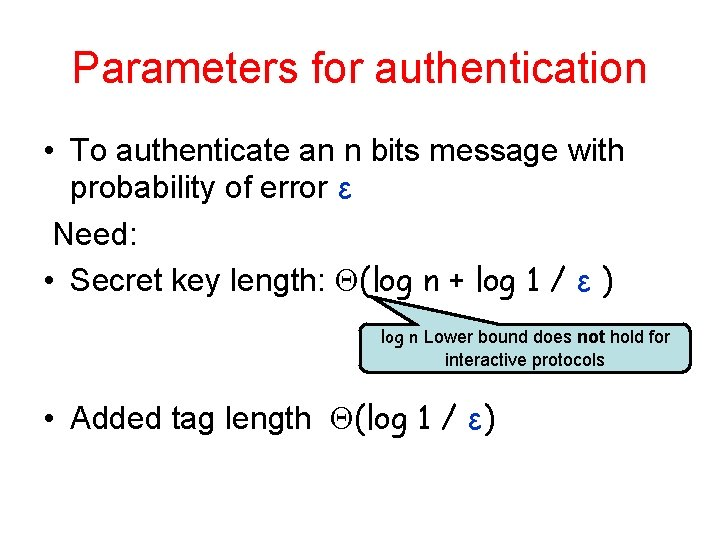Parameters for authentication • To authenticate an n bits message with probability of error ε Need: • Secret key length: (log n + log 1 / ε ) log n Lower bound does not hold for interactive protocols • Added tag length (log 1 / ε)Authentication for Storage • Large file residing on a remote server • Verifier stores a small secret `representative’ of file – Fingerprint – When retrieving the file should identify corruption • The size of the fingerprint – A well understood problem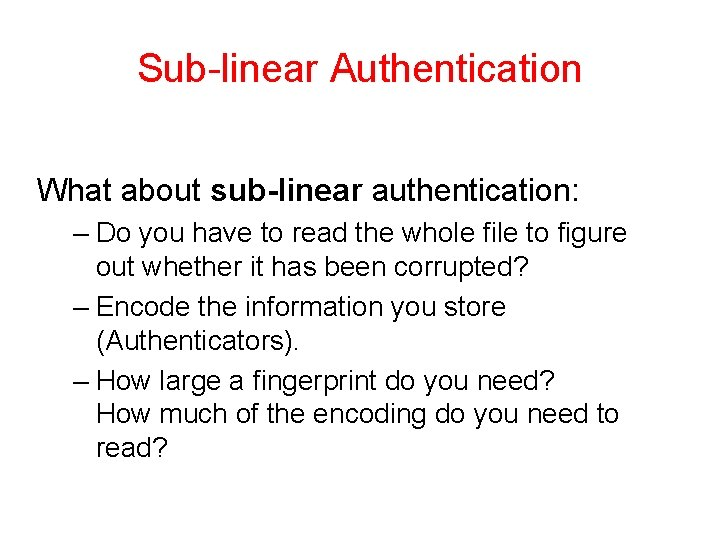Sub-linear Authentication What about sub-linear authentication: – Do you have to read the whole file to figure out whether it has been corrupted? – Encode the information you store (Authenticators). – How large a fingerprint do you need? How much of the encoding do you need to read?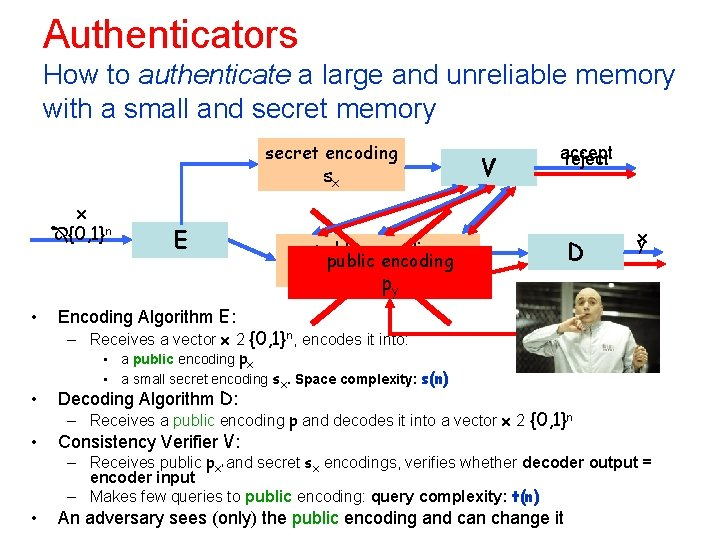Authenticators How to authenticate a large and unreliable memory with a small and secret memory secret encoding sx x {0, 1}n E V accept reject public encoding px py • Encoding Algorithm E: • Decoding Algorithm D: • Consistency Verifier V: • An adversary sees (only) the public encoding and can change it D x y – Receives a vector x 2 {0, 1}n, encodes it into: • a public encoding px • a small secret encoding sx. Space complexity: s(n) – Receives a public encoding p and decodes it into a vector x 2 {0, 1}n – Receives public px’ and secret sx encodings, verifies whether decoder output = encoder input – Makes few queries to public encoding: query complexity: t(n)Power of the Adversary • We have seen the access the Adversary has to the system • Distinguish between computationally – all powerful and – Bounded Adversaries Dr. Evil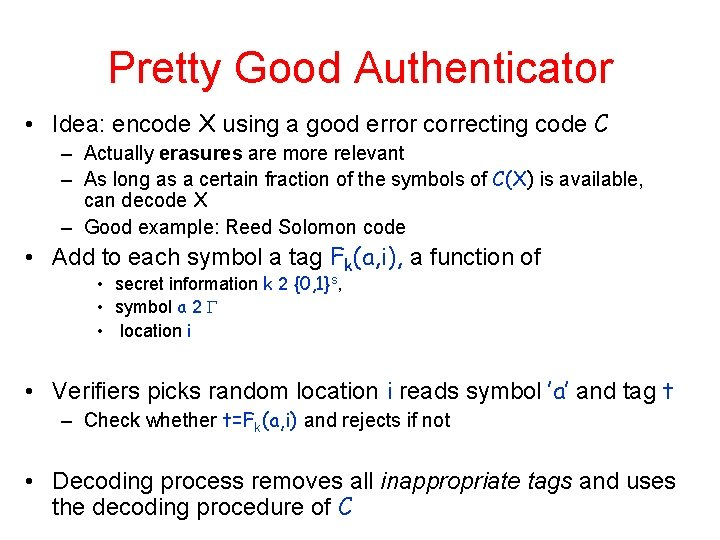Pretty Good Authenticator • Idea: encode X using a good error correcting code C – Actually erasures are more relevant – As long as a certain fraction of the symbols of C(X) is available, can decode X – Good example: Reed Solomon code • Add to each symbol a tag Fk(a, i), a function of • secret information k 2 {0, 1}s, • symbol a 2 • location i • Verifiers picks random location i reads symbol ’a’ and tag t – Check whether t=Fk(a, i) and rejects if not • Decoding process removes all inappropriate tags and uses the decoding procedure of C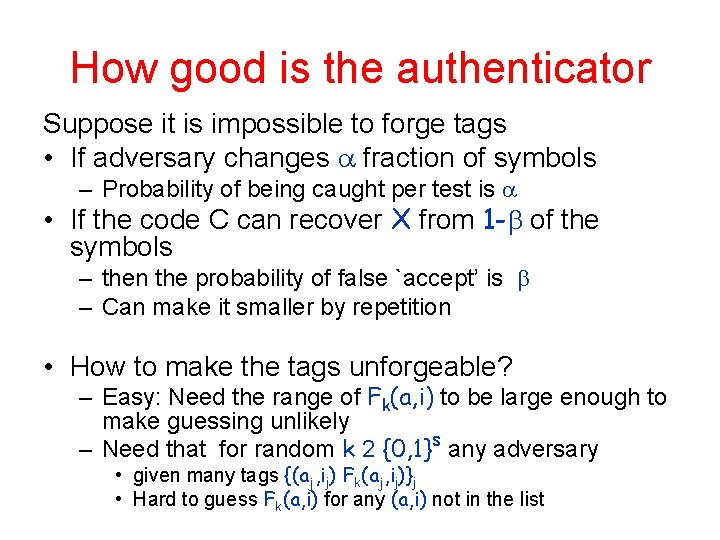How good is the authenticator Suppose it is impossible to forge tags • If adversary changes fraction of symbols – Probability of being caught per test is • If the code C can recover X from 1 - of the symbols – then the probability of false `accept’ is – Can make it smaller by repetition • How to make the tags unforgeable? – Easy: Need the range of Fk(a, i) to be large enough to make guessing unlikely – Need that for random k 2 {0, 1}s any adversary • given many tags {(aj, ij) Fk(aj, ij)}j • Hard to guess Fk(a, i) for any (a, i) not in the list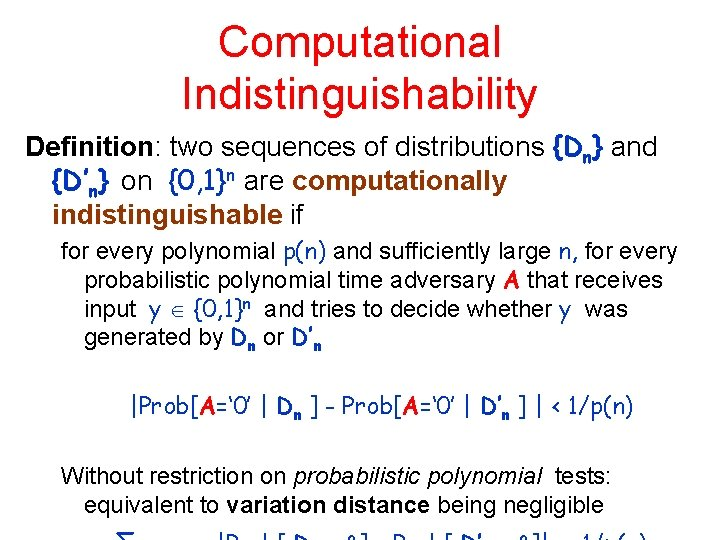Computational Indistinguishability Definition: two sequences of distributions {Dn} and {D’n} on {0, 1}n are computationally indistinguishable if for every polynomial p(n) and sufficiently large n, for every probabilistic polynomial time adversary A that receives input y {0, 1}n and tries to decide whether y was generated by Dn or D’n |Prob[A=‘ 0’ | Dn ] - Prob[A=‘ 0’ | D’n ] | < 1/p(n) Without restriction on probabilistic polynomial tests: equivalent to variation distance being negligiblePseudo-random Functions Let {s(n), m(n), ℓ(n)} be a sequence of parameters: F: {0, 1}s {0, 1}m {0, 1}ℓ key Domain Range Denote Y= Fk (X) A family of functions Φn ={Fk | k 0, 1}s } is pseudo-random if it is • Efficiently computable - random access and. . .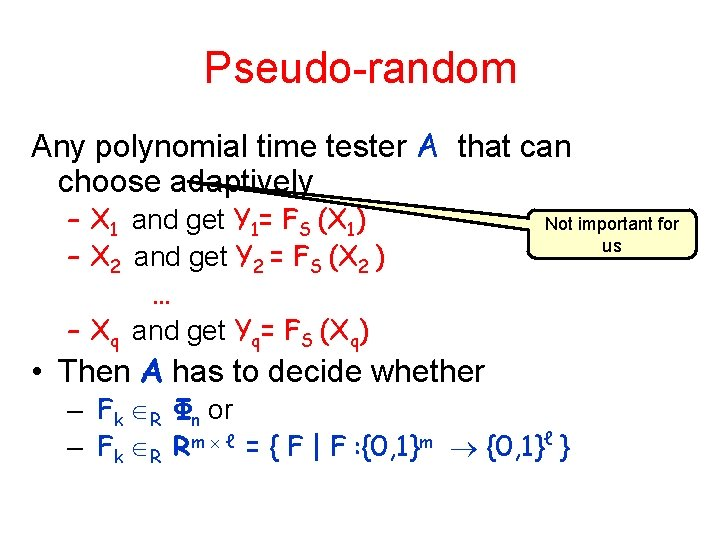Pseudo-random Any polynomial time tester A that can choose adaptively – X 1 and get Y 1= FS (X 1) – X 2 and get Y 2 = FS (X 2 ) … – Xq and get Yq= FS (Xq) • Then A has to decide whether Not important for us – Fk R Φn or – Fk R Rm ℓ = { F | F : {0, 1}m {0, 1}ℓ }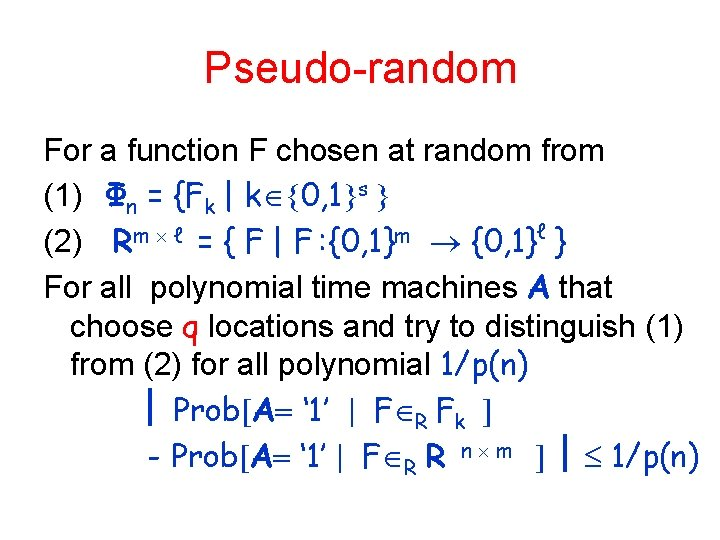Pseudo-random For a function F chosen at random from (1) Φn = {Fk | k 0, 1 s ℓ m (2) R = { F | F : {0, 1} } For all polynomial time machines A that choose q locations and try to distinguish (1) from (2) for all polynomial 1/p(n) Prob A ‘ 1’ F R Fk - Prob A ‘ 1’ F R R n m 1/p(n)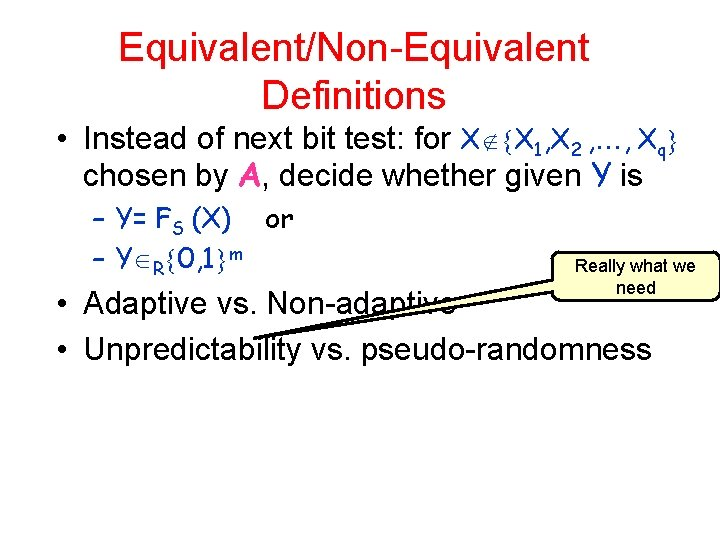Equivalent/Non-Equivalent Definitions • Instead of next bit test: for X X 1, X 2 , , Xq chosen by A, decide whether given Y is – Y= FS (X) or – Y R 0, 1 m Really what we need • Adaptive vs. Non-adaptive • Unpredictability vs. pseudo-randomnessExistence of Pseudo-Random functions and Authenticators • If one-way functions exist so pseudorandom generators • If pseudo-random generators exist, so do Probability of pseudo-random functions error Authenticators • Conclusion: If one-way functions exist, – so do sublinear authenticators with • Secret memory: sufficient to store a key • Query complexity log n or log n log 1/So are we done Two problems: • Need – computational bounded adversary and – one-way functions • Efficiency: the evaluation of a pseudorandom function might be a burden to add to every memory fetch operationCommunication Complexity x 2 X Alice Bob y 2 Y Let f: X x Y Z Input is split between two participants Want to compute outcome: z=f(x, y) while exchanging as few bits as possible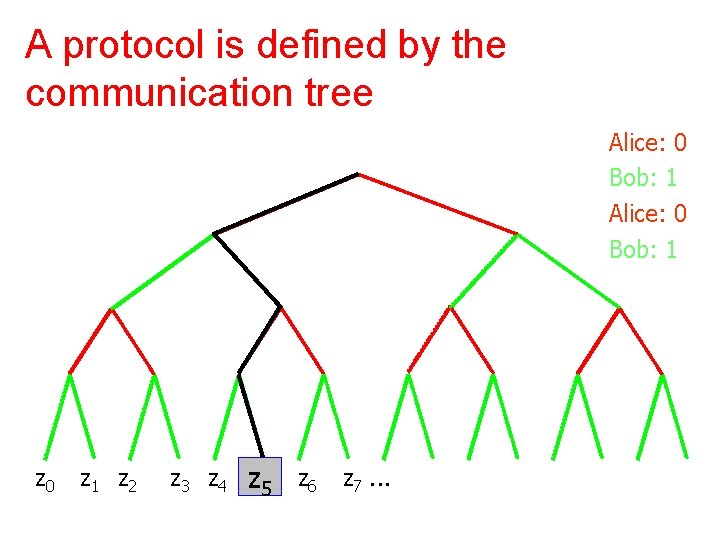A protocol is defined by the communication tree Alice: 0 Bob: 1 z 0 z 1 z 2 z 3 z 4 zz 55 z 6 z 7. . .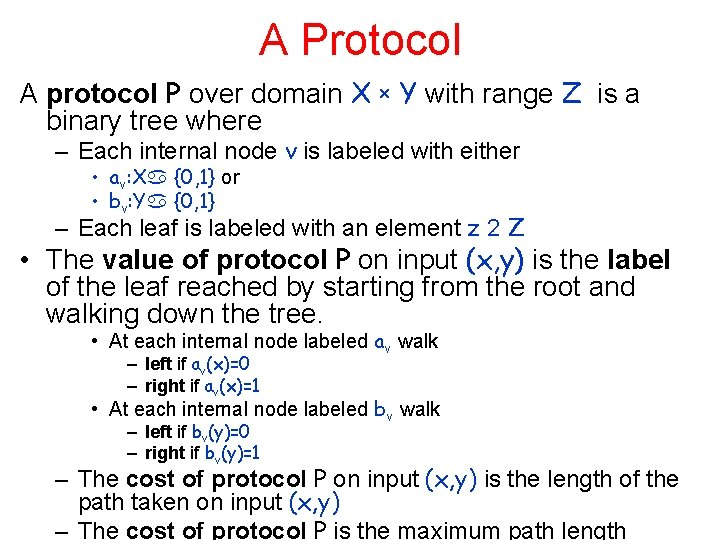A Protocol A protocol P over domain X x Y with range Z is a binary tree where – Each internal node v is labeled with either • av: X {0, 1} or • bv: Y {0, 1} – Each leaf is labeled with an element z 2 Z • The value of protocol P on input (x, y) is the label of the leaf reached by starting from the root and walking down the tree. • At each internal node labeled av walk – left if av(x)=0 – right if av(x)=1 • At each internal node labeled bv walk – left if bv(y)=0 – right if bv(y)=1 – The cost of protocol P on input (x, y) is the length of the path taken on input (x, y) – The cost of protocol P is the maximum path length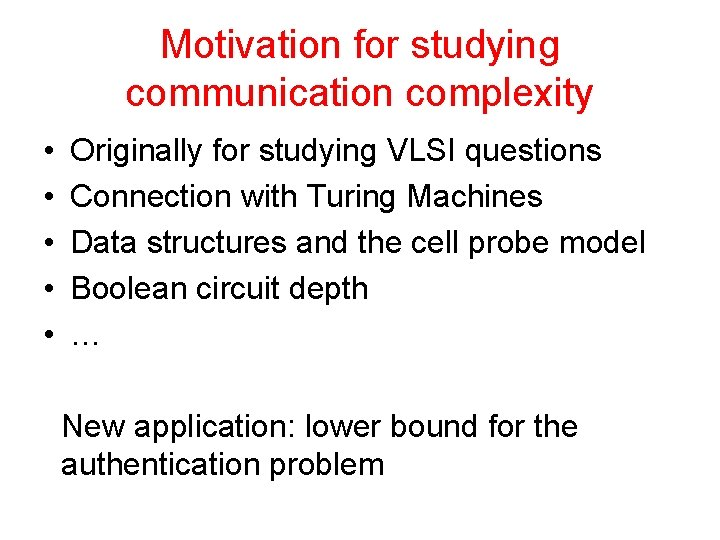Motivation for studying communication complexity • • • Originally for studying VLSI questions Connection with Turing Machines Data structures and the cell probe model Boolean circuit depth … New application: lower bound for the authentication problemCommunication Complexity of a function • For a function f: X x Y Z the (deterministic) communication complexity of f (D(f)) is the minimum cost of protocol P over all protocols that compute f Observation: For any function f: X x Y Z D(f) ≤ log |X| + log |Z| Example: let x, y µ {1, …, n} and let f(x, y)=max{x [ y} Then D(f) · 2 log nMedian let x, y µ {1, …, n} and let MED(x, y) be the median of the multiset x [ y If the size is even then element ranked |x[ y|/2 Claim: D(MED) is O(log 2 n) protocol idea: do a binary search on the value, each party reporting how many are above the current guess Exercise: D(MED) is O(log n) protocol idea: each party proposes a candidate See which one is larger - no need to repeat bits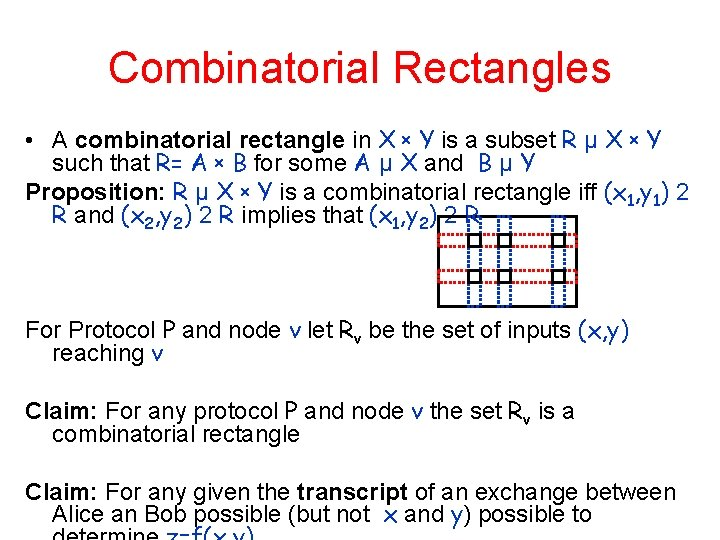Combinatorial Rectangles • A combinatorial rectangle in X x Y is a subset R µ X x Y such that R= A x B for some A µ X and B µ Y Proposition: R µ X x Y is a combinatorial rectangle iff (x 1, y 1) 2 R and (x 2, y 2) 2 R implies that (x 1, y 2) 2 R For Protocol P and node v let Rv be the set of inputs (x, y) reaching v Claim: For any protocol P and node v the set Rv is a combinatorial rectangle Claim: For any given the transcript of an exchange between Alice an Bob possible (but not x and y) possible toFooling Sets • For f: X x Y Z a subset R µ X x Y is f-monochromatic if f is fixed on R • Observation: any protocol P induces a partition of X x Y into f-monochromatic rectangles. The number of rectangles is the number of leaves in P • A set Sµ X x Y is a fooling set for f if there exists a z 2 Z where – For every (x, y) 2 S, f(x, y)=z – For every distinct (x 1, y 1), (x 2, y 2) 2 S either • f(x 1, y 2)≠z or • f(x 2, y 1)≠z y 1 x 2 y 2 z z Property: no two elements of a fooling set S can be in the same monochromatic rectangle Lemma: if f has a fooling set of size t, then D(f) ≥ log 2 tApplications Equality: Alice and Bob each hold x, y 2 {0, 1}n – want to decide whether x=y or not. • Fooling set for Equality S={(w, w)|w 2 {0, 1}n } Conclusion: D(Equality) ¸ n Disjointness: let x, y µ {1, …, n} and let – DISJ(x, y)=1 if |x y|¸ 1 and – DISJ(x, y)=0 otherwise • Fooling set for Disjointness S={(A, comp(A))|A µ {1, …, n} } Conclusion: D(DISJ) ¸ nProbabilistic Protocols Random coins x y ALICE BOB• Probabilistic Communication Complexity Alice an Bob have each, in addition to their inputs, access to random strings of arbitrary length r. A and r. B (respectively) A probabilistic protocol P over domain X x Y with range Z is a binary tree where – Each internal node v is labeled with either av(x, r. A ) or bv(y, r. B ) – Each leaf is labeled with an element z 2 Z Take all probabilities over the choice of r. A and r. B • P computes f with zero error if for all (x, y) Pr[P(x, y)=f(x, y)]=1 • P computes f with error if for all (x, y) Pr[P(x, y)=f(x, y)]¸ 1 - • For Boolean f, P computes f with one-sided error if for all (x, y) s. t. f(x, y)=0 Pr[P(x, y)=0]=1 and for all (x, y) s. t. f(x, y)=1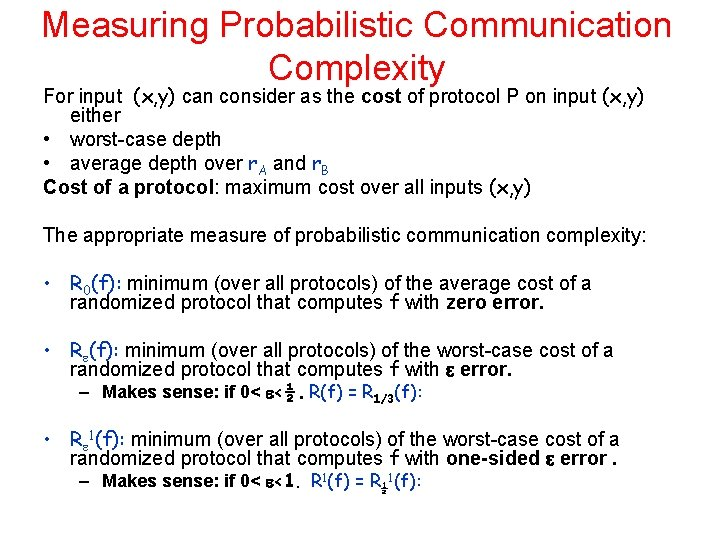Measuring Probabilistic Communication Complexity For input (x, y) can consider as the cost of protocol P on input (x, y) either • worst-case depth • average depth over r. A and r. B Cost of a protocol: maximum cost over all inputs (x, y) The appropriate measure of probabilistic communication complexity: • R 0(f): minimum (over all protocols) of the average cost of a randomized protocol that computes f with zero error. • R (f): minimum (over all protocols) of the worst-case cost of a randomized protocol that computes f with error. – Makes sense: if 0< <½. R(f) = R 1/3(f): • R 1(f): minimum (over all protocols) of the worst-case cost of a randomized protocol that computes f with one-sided error. – Makes sense: if 0< <1. R 1(f) = R½ 1(f):Equality • Idea: pick a family of hash functions H={h|h: {0, 1}n {1…m}} such that for all x≠y, for random h 2 RH Pr[(h(x)=h(y)]· Protocol: Alice: pick random h 2 RH and send <h, h(x)> Bob: compare h(x) to h(y) and announce the result This is a one-sided error protocol with cost log|H|+ log m Constructing H: Fact: over any two polynomials of degree d agree on at most d points Fix prime q such that n 2 · q · 2 n 2 map x to a polynomial Wx of degree d=n/log q over GF[q] H={hz|z 2 GF[q]} and hz(x)=Wx(z) = d/q= n/q log q · 1/n log nPublic coins model • What if Alice and Bob have access to a joint source of bits. Possible view: distribution over deterministic protocols Let R pub(f): be the minimum cost of a public coins protocol computing f correctly with probability at least 1 - for any input (x, y) Example: R pub(Equality) = (-log ) Theorem: for any Boolean f: R + (f) is R pub(f)+O(log n + log 1/ ) Proof: choose t = 8 n/ 2 assignments to the public string…Simulating large sample spaces Collection that should resemble probability of success on ALL inputs Bad Good 1 - • Want to find among all possible public random strings a small collection of strings on which the protocol behave similarly on all inputs • Choose m random strings • For input (x, y) event Ax, y is more than ( + ) of the m strings fail the protocol 2 t -2 Pr[Ax, y] · e < 2 -2 n Pr[[x, y A x, y] · x, y Pr[A 2 n 2 -2 n=1 ] <2 x, y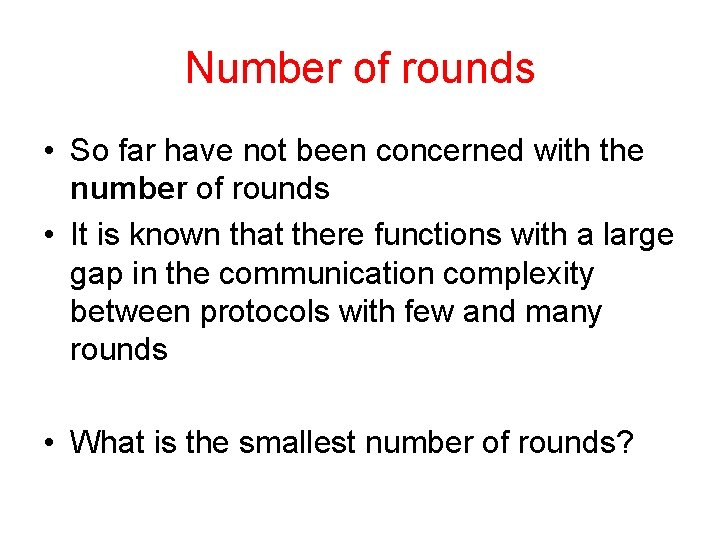Number of rounds • So far have not been concerned with the number of rounds • It is known that there functions with a large gap in the communication complexity between protocols with few and many rounds • What is the smallest number of rounds?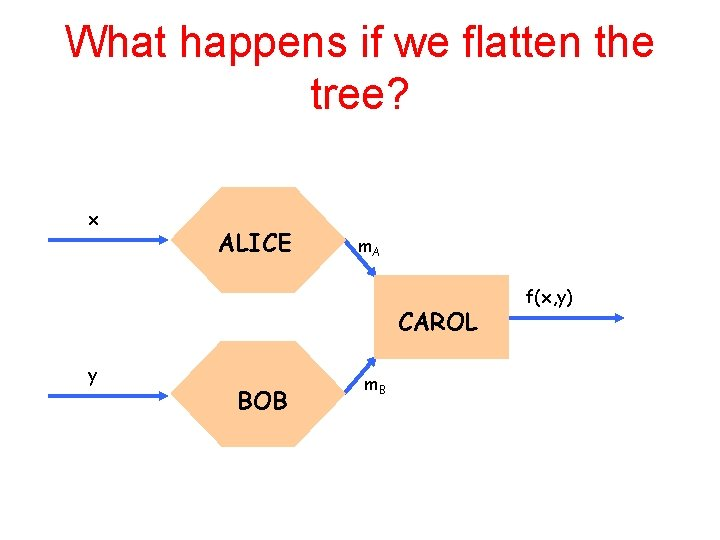What happens if we flatten the tree? x ALICE m. A CAROL y BOB m. B f(x, y)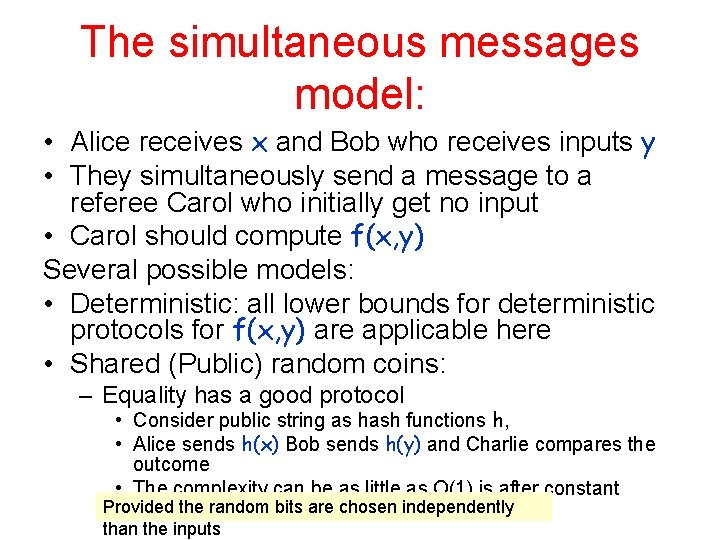The simultaneous messages model: • Alice receives x and Bob who receives inputs y • They simultaneously send a message to a referee Carol who initially get no input • Carol should compute f(x, y) Several possible models: • Deterministic: all lower bounds for deterministic protocols for f(x, y) are applicable here • Shared (Public) random coins: – Equality has a good protocol • Consider public string as hash functions h, • Alice sends h(x) Bob sends h(y) and Charlie compares the outcome • The complexity can be as little as O(1) is after constant Provided the random bits are chosen independently probability of error than the inputs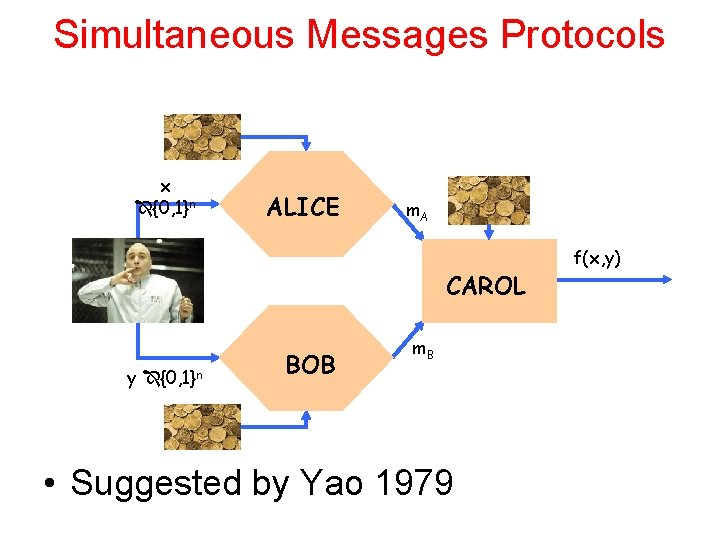Simultaneous Messages Protocols x {0, 1}n ALICE m. A CAROL y {0, 1}n BOB m. B • Suggested by Yao 1979 f(x, y)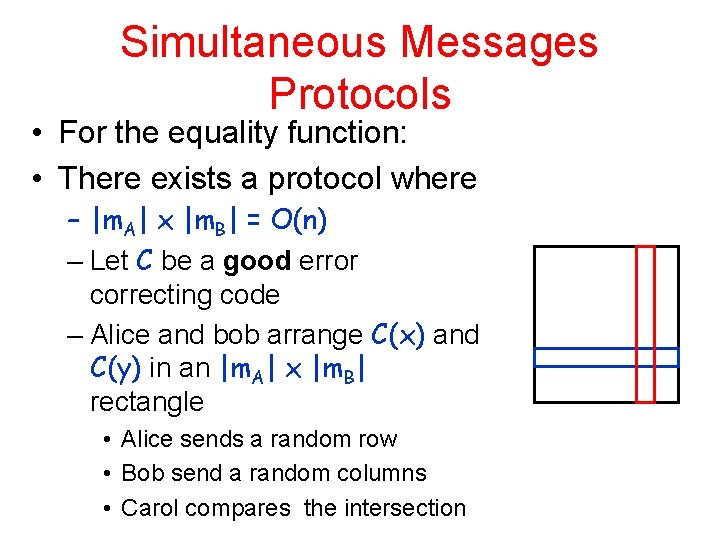Simultaneous Messages Protocols • For the equality function: • There exists a protocol where – |m. A| x |m. B| = O(n) – Let C be a good error correcting code – Alice and bob arrange C(x) and C(y) in an |m. A| x |m. B| rectangle • Alice sends a random row • Bob send a random columns • Carol compares the intersection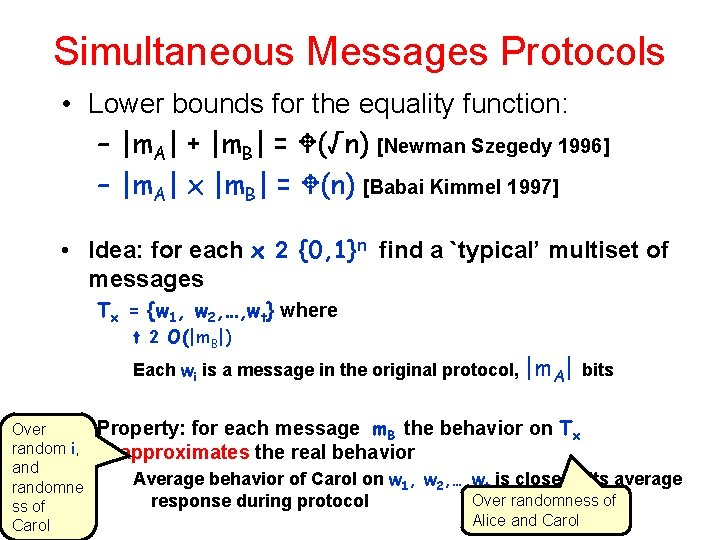Simultaneous Messages Protocols • Lower bounds for the equality function: – |m. A| + |m. B| = (√n) [Newman Szegedy 1996] – |m. A| x |m. B| = (n) [Babai Kimmel 1997] • Idea: for each x 2 {0, 1}n find a `typical’ multiset of messages Tx = {w 1, w 2, …, wt} where t 2 O(|m. B|) Each wi is a message in the original protocol, |m. A| bits Over random i, and randomne ss of Carol Property: for each message m. B the behavior on Tx approximates the real behavior Average behavior of Carol on w 1, w 2, …, wt is close to its average Over randomness of response during protocol Alice and CarolSimultaneous Messages Protocols How to find for each x 2 {0, 1} such a `typical’ T n size t • Claim: a random choice of wi’s is good Proof by Chernoff – Need to `take care’ of every m. B (2|m. B| possibilities ) • Claim: for x x’ we have Tx Tx’ – Otherwise behaves the same when y = x for x and x’ • Let Sx be the m. B’s for which protocol mostly says ’ 1’ • Let Wx be the m. B’s for which protocol mostly says ’ 0’ • Then for y=x the distribution should be mostly on Sx • Conclusion: t ¢ |m. A| ¸ n and we get |m. A| x |m. B| = (n) [Babai Kimmel 1997] x of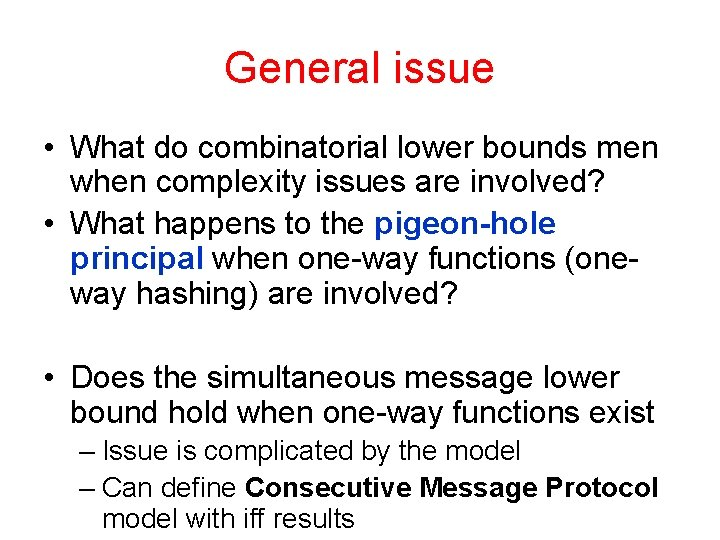General issue • What do combinatorial lower bounds men when complexity issues are involved? • What happens to the pigeon-hole principal when one-way functions (oneway hashing) are involved? • Does the simultaneous message lower bound hold when one-way functions exist – Issue is complicated by the model – Can define Consecutive Message Protocol model with iff resultsAnd now for something completely different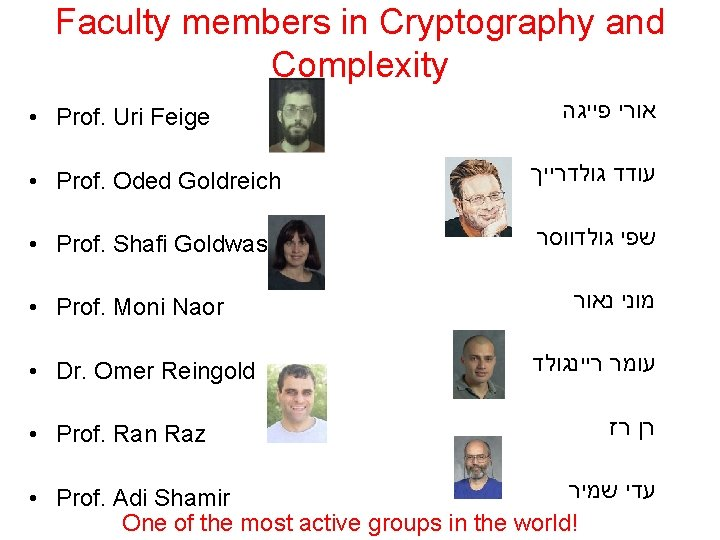Faculty members in Cryptography and Complexity • Prof. Uri Feige אורי פייגה • Prof. Oded Goldreich עודד גולדרייך • Prof. Shafi Goldwasser שפי גולדווסר • Prof. Moni Naor • Dr. Omer Reingold • Prof. Ran Raz מוני נאור עומר ריינגולד רן רז עדי שמיר • Prof. Adi Shamir One of the most active groups in the world!